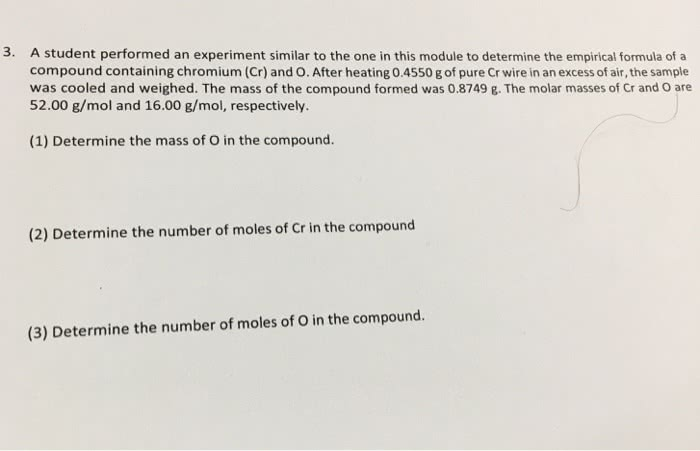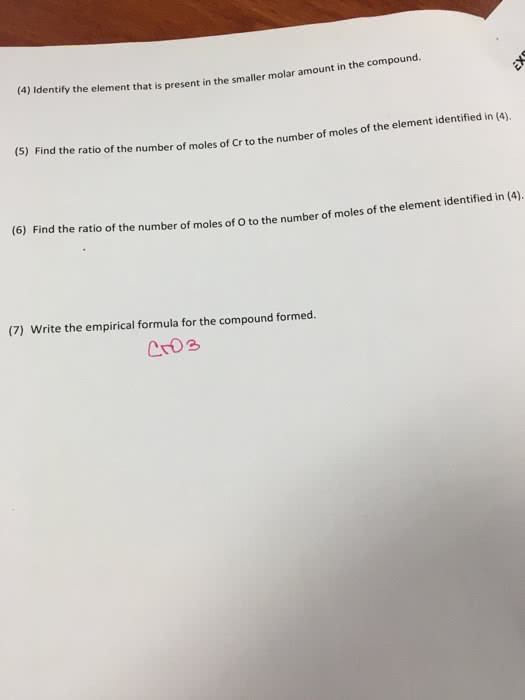1
0
watching
410
views11 Nov 2019

#3. A student performed an experiment similar to the one in this module to determine the empirical formula of a compound containing chromium (Cr) and O. After heating 0.4550 g of pure Cr wire in an excess of air, the sample was cooled and weighed. The mass of the compound formed was 0.8749 g. The molar masses of Cr and O are 52.00 g/mol and 16.00 g/mol, respectively. (1) Determine the mass of O in the compound. (2) Determine the number of moles of Cr in the compound (3) Determine the number of moles of O in the compound